# Arrays in Arduino  What are they? + How to Use ▷ 2021

Arduino boards are characterized for having one fairly simple programming in IDE environment. This is due to the use of Arrays and Strings, which allow to simplify a set of variables and diverse data.

If you want to get inside world of Arduino programming it is important that you know these two functions because they will help you save time no longer make mistakes.

To know more, it is important that you continue reading until the end. We will explain what these concepts are about and the step by step you must do to use a matrix. Let’s get started.

## What is an Array in Arduino programming and what are they for?

We will show you below what two very important elements are used in programming an Arduino board:

### Arrays

Arrays, vectors, or matrices, are containers for variables. That is, they are “Variables” that contain others to be able to group them. They are also called matrices, since its layout represented graphically is similar to that of a matrix, and are also called vectors because the arrays of a single row or column are given the same name.

To be able to use an array, there are two requirements. The first is that all the contained variables are of the same nature and the second is, that the limit of variables that the program can save is not exceeded.

This limit varies according to the array, since we predefine it ourselves at the time of declaring it (it is important that, when we create an array, we reserve memory that is used to define the end of the array). We can say then that, it is a set of variables gathered under a single name, but that within this group there may be other different ones, of the same type and ordered by an index. This makes programming easier as it makes the code simpler and clearer.

### Strings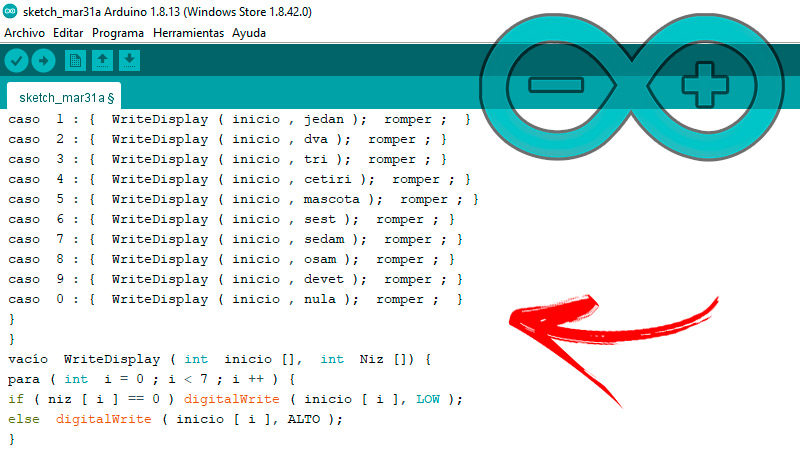Strings of characters or “Strings” they are data types that allow to gather diverse characters. They differ from the “char” because they allow you to save more than a single character. it’s possible store words, phrases, numbers, special characters or the composition of these elements.

For instance:

```String str1 = "bienvenido";

String str2 = "bienvenido aspirante";

String str3 = "54321";

String str4 = "bienvenido usuario 54321";

String str5 = "*/-+";

String str6 = "bienvenido usuario ++**54325**++";
```

Thanks to this tool it is feasible to obtain different results in a simpler way. Among them is concatenation, which consists of assembling two character strings into one.

It is of great help when you want to make code sequences, see below:

```String str1 = "bienvenido";

String str2 = "usuario";

String str3 = "";

str3 += str1;

str3 += " ";

str3 += str2;
```

If “str3” is printed the result will give “welcome user”. What we did was create a concatenation of the value “Welcome” plus the variable “Username”, after indicating a empty String variable. Another of the utilities it has are “ToUpperCase ()” and “ToLowerCase ()”, which replaces all characters in the string with uppercase and lowercase, respectively. It is also very practical for converting numbers from the decimal system to Hexa, octal or binary.

We will use 26 as an example:

```int num = 26;

String hex = String(num, HEX);

String bin = String(num, BIN);

String oct = String(num, OCT);

println(hex);

println(bin);

println(oct);

Al imprimir los resultados darán

1A

11010

32
```

1A would be 26 in hexadecimal, while 11010 is the result in binary and 32 in octal system.

## Learn step by step how to use a matrix or Array when programming with Arduino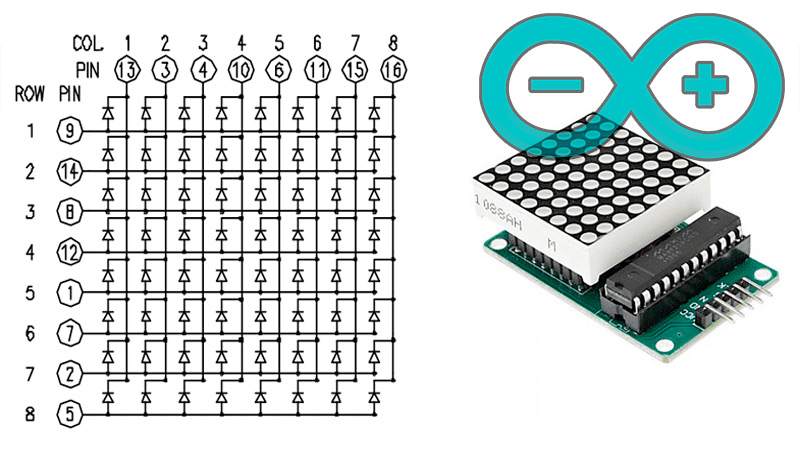Both arrays and strings they are indispensable tools that you must learn to use if you want to program your Arduino board.

For this we leave you these basic indications to get you started:

### To declare

It is important clarify that to use arrays, the first thing you have to do is index the first element with the number zero. In this way we can already consult it and assign values ​​to it.

We can declare them in any of the following ways:

```int losEnteros; //declara una matriz de 6 variables de tipo int

int losPins[] = {2, 4, 8, 3}; //declara una matriz de 4 variables tipo int

float losSensores = {2, 4, -8, 3.8, 2.1, 6}; //declara una matriz de 6 variables tipo float

char mensaje = "Saludo"; //declara una matriz de 5 elementos tipo char

char mensaje  = {'s','a','l','u','d','o'}; //declara una matriz de 6 elementos tipo char

int mimatriz;

int Pines[] = {2, 4, 8, 3, 6};

int losValores = {2, 4, -8, 3, 2};
```

You must bear in mind that char arrays require an extra character to identify their nial.

### Access an array

The matrix, as we already said, is indexed from scratch, so the first value is at index 0. According to the example, the values will be equal to 2 and the Values will be 4. Therefore we must be careful when accessing the matrix. If we try to access beyond the end (using an index less than zero or greater than the declared size) the memory locations used for other purposes will cause errors.

### Assign a value to an array

In turn, to assign the values ​​of an array, the assigning operator (=) is used, continuing with the example as follows:

• `losSensores = 10; //asigna 10 al primer elemento de la matriz`
• `x = losSensores; //guarda el valor del quinto elemento de la matriz en la variable x`

### Retrieve a value from an array

To retrieve the specific value of a specific array and according to the example, we only have to use the line:

## List of the best Arduino projects with Arrays that you can do to practice

Now what do you know what these tools are about, the time has come to put them into practice.

For this we leave you these three projects so that you can exercise and put into practice everything you have learned in this post:

### Memory game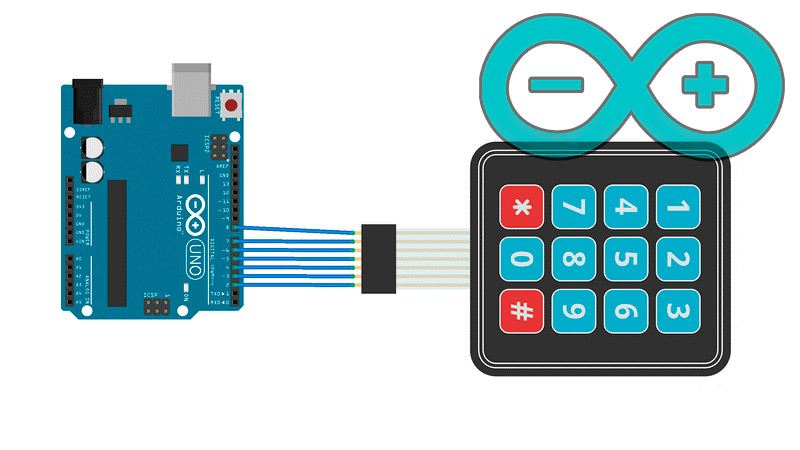It is a playful application in which we must memorize as many digits as possible until the loading of the “drums”.

As for the hardware we will need:

• Arduino Mega 2560
• A 7 segment display (similar to the digits on calculators).
• A 3×4 type Arduino keypad
• Generic jumpers
• A mini battery charge display

Then we connect them to each other as follows: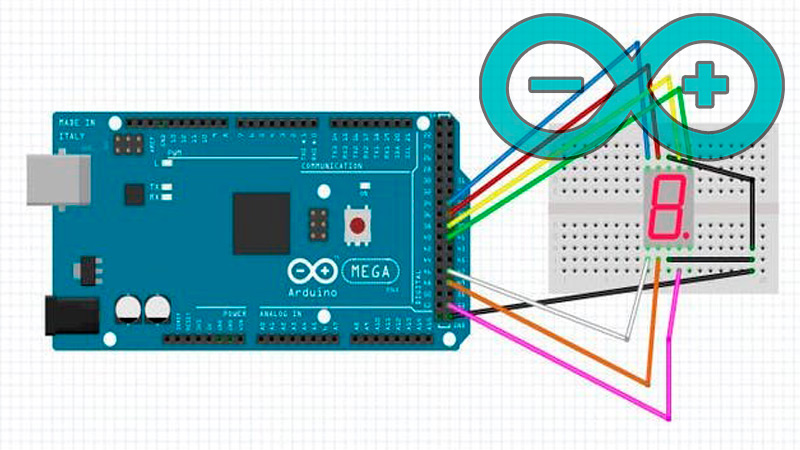• The first pin from the left side to pin 8 of the Arduino. Pins 8 to 2 on the Arduino Mega control unit. According to the picture.
• The seven segment display connects to pins to pins 35,37,39,41,47,49 and 53.
• The mini battery display connects via pins DIO at 9 and CLK at 10.

Regarding the software, this is the code:

```#include <Teclado.h>

#include <TM1651.h>

int CLK  =  10 ;

int DIO  =  9 ;

Pantalla de batería TM1651 ( CLK , DIO );

const byte  FILAS  =  4 ;

const byte  COLS  =  3 ;

char claves [ FILAS ] [ COLS ]  =  {

{ '1' , '2' , '3' },

{ '4' , '5' , '6' },

{ '7' , '8' , '9' },

{ '*' , '0' , "https://internetpasoapaso.com/arrays-arduino/#" }

};

byte rowPins [ FILAS ]  =  { 8 ,  7 ,  6 ,  5 };

byte colPins [ COLS ]  =  { 4 ,  3 ,  2 };

Teclado teclado  =  Teclado (  makeKeymap ( teclas ),  rowPins ,  colPins ,  FILAS ,  COLS  );

int inicio [ 7 ] = { 39 , 41 , 53 , 49 , 47 , 37 , 35 };

int ugasi [ 7 ] = { 0 , 0 , 0 , 0 , 0 , 0 , 0 };

int jedan [ 7 ] = { 0 , 1 , 1 , 0 , 0 , 0 , 0 };

En t dva [ 7 ] = { 1 , 1 , 0 , 1 , 1 , 0 , 1 };

int tri [ 7 ] = { 1 , 1 , 1 , 1 , 0 , 0 , 1 };

int cetiri [ 7 ] = { 0 , 1 , 1 , 0 , 0 , 1 , 1 };

mascota int [ 7 ] = { 1 , 0 , 1 , 1 , 0 , 1 , 1 };

int sest [ 7 ] = { 0 , 0 , 1 , 1 , 1 , 1 , 1 };

int sedam [ 7 ] = { 1 , 1 , 1 , 0 , 0 , 0 , 0 };

int osam [ 7 ] = { 1 , 1 , 1 , 1 , 1 , 1 , 1 };

int devet [ 7 ] = { 1 , 1 , 1 , 0 , 0 , 1 , 1 };

int nula [ 7 ] = { 1 , 1 , 1 , 1 , 1 , 1 , 0 };

int pom = 0 ;

int s [ 50 ];

int k = 0 ;

int partija = 0 ;

configuración vacía () {

Serial . comenzar ( 9600 );

Salida ( inicio );

BatteryDisplay . init ();

BatteryDisplay . establecer ( MÁS BRILLANTE ); // BRIGHT_TYPICAL = 2, BRIGHT_DARKEST = 0, BRIGHTEST = 7;

BatteryDisplay . marco ( FRAME_ON );

randomSeed ( analogRead ( 0 ));

while ( partija ! = 8 ) {

BatteryDisplay . displayLevel ( partija );

s [ k ] = aleatorio ( 0 , 10 );

WriteDisplay ( inicio , ugasi );

retraso ( 200 );

ispisi ( s [ k ]);

if ( Konacno ( k ) ! = 0 ) {

WriteDisplay( inicio , ugasi );

romper ;

}

partija ++ ; k ++ ;

} // mientras finaliza

} // finalización de la configuración

vacío bucle () {}

int Konacno ( int  k ) {

int pom= 0 ;

mientras ( pom <= k ) {

char key  =  teclado . getKey ();

while ( ! tecla ) { tecla  =  teclado . obtener la clave ();  }

if ( s [ pom ] ! = K ( tecla )) return  - 1 ;

pom ++ ;

retraso ( 400 );

}

return 0 ;

} // fin de konacno

int K ( Char  clave ) {

if ( key == '1' ) return 1 ;

else if ( clave == '2' ) return  2 ;

else if ( clave == '3' ) return  3 ;

else if ( clave == '4' ) return  4 ;

else if ( clave == '5' ) return  5 ;

else if ( clave == '6' ) volver  6 ;

else if ( clave == '7' ) return  7 ;

else if ( clave == '8' ) return  8 ;

else if ( clave == '9' ) return  9 ;

}

}```

### Knight Rider, the fantastic car or the fantastic car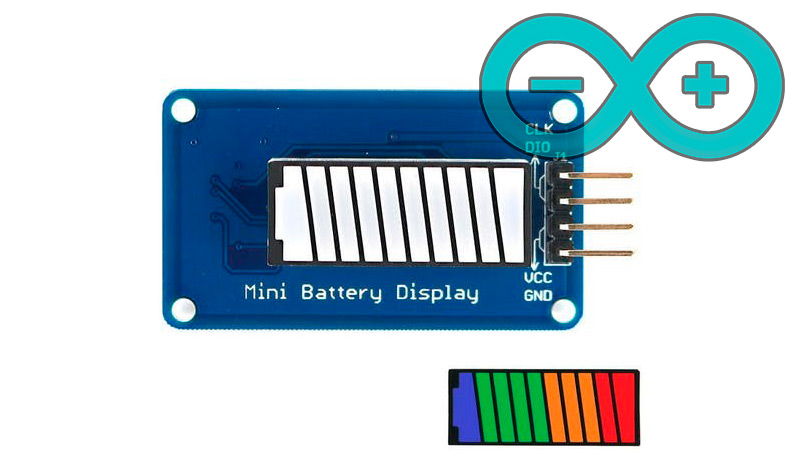If these names do not sound like you, surely your parents or even grandparents. It is a code that makes a series of LEDs behave in the same way that one of the main characters in this series of the eighties did.

We will need:

• 6 LED
• 6 220 Ohm resistors
• Arduino UNO board
• Generic jumpers

The LEDs are connected to pins 2 to 7 using a resistor in each jumper. We will also show you 3 different ways to program this visual effect. In the first we will only use “DigitalWrite” (pinNum, HIGH / LOW) and “delay” (time). In the second we will do it through the construction of the type “For” which will do the same, but in code with fewer lines.

Finally, the code that will perform this effect more smoothly:

```/* Knight Rider 1

*/

int pin2 = 2;

int pin3 = 3;

int pin4 = 4;

int pin5 = 5;

int pin6 = 6;

int pin7 = 7;

int timer = 100;

void setup(){

pinMode(pin2, OUTPUT);

pinMode(pin3, OUTPUT);

pinMode(pin4, OUTPUT);

pinMode(pin5, OUTPUT);

pinMode(pin6, OUTPUT);

pinMode(pin7, OUTPUT);

}

void loop() {

digitalWrite(pin2, HIGH);

delay(timer);

digitalWrite(pin2, LOW);

delay(timer);

digitalWrite(pin3, HIGH);

delay(timer);

digitalWrite(pin3, LOW);

delay(timer);

digitalWrite(pin4, HIGH);

delay(timer);

digitalWrite(pin4, LOW);

delay(timer);

digitalWrite(pin5, HIGH);

delay(timer);

digitalWrite(pin5, LOW);

delay(timer);

digitalWrite(pin6, HIGH);

delay(timer);

digitalWrite(pin6, LOW);

delay(timer);

digitalWrite(pin7, HIGH);

delay(timer);

digitalWrite(pin7, LOW);

delay(timer);

digitalWrite(pin6, HIGH);

delay(timer);

digitalWrite(pin6, LOW);

delay(timer);

digitalWrite(pin5, HIGH);

delay(timer);

digitalWrite(pin5, LOW);

delay(timer);

digitalWrite(pin4, HIGH);

delay(timer);

digitalWrite(pin4, LOW);

delay(timer);

digitalWrite(pin3, HIGH);

delay(timer);

digitalWrite(pin3, LOW);

delay(timer);

}

/* Knight Rider 2

*/

int pinArray[] = {2, 3, 4, 5, 6, 7};

int count = 0;

int timer = 100;

void setup(){

// we make all the declarations at once

for (count=0;count<6;count++) {

pinMode(pinArray[count], OUTPUT);

}

}

void loop() {

for (count=0;count<6;count++) {

digitalWrite(pinArray[count], HIGH);

delay(timer);

digitalWrite(pinArray[count], LOW);

delay(timer);

}

for (count=5;count>=0;count--) {

digitalWrite(pinArray[count], HIGH);

delay(timer);

digitalWrite(pinArray[count], LOW);

delay(timer);

}

}

/* Knight Rider 3

*/

int pinArray[] = {2, 3, 4, 5, 6, 7};

int count = 0;

int timer = 30;

void setup(){

for (count=0;count<6;count++) {

pinMode(pinArray[count], OUTPUT);

}

}

void loop() {

for (count=0;count<5;count++) {

digitalWrite(pinArray[count], HIGH);

delay(timer);

digitalWrite(pinArray[count + 1], HIGH);

delay(timer);

digitalWrite(pinArray[count], LOW);

delay(timer*2);

}

for (count=5;count>0;count--) {

digitalWrite(pinArray[count], HIGH);

delay(timer);

digitalWrite(pinArray[count - 1], HIGH);

delay(timer);

digitalWrite(pinArray[count], LOW);

delay(timer*2);

}

}```

### Binary Clock

A binary clock it is an artifact that visualizes time (which is sexagesimal) in a compound binary expression, i.e. six digits (2 for the hour, 2 for the minutes and 2 for the seconds).

It is really easy to do, to start we will need:

• 1 Breadboard
• 6 LEDs Color Red
• 6 LEDs Color Green
• 5 leds Color Yellow
• 17 330 ohm resistors
• 17 jumpers
• Arduino UNO

With everything learned, you will be able to elucidate all the arrays that are presented in the necessary code to operate this watch.

Check out:

```int ledPinsSec[] = {2, 3, 4, 5, 6, 7};

int ledPinsMin[] = {8, 9, 10, 11, 12, 13};

int ledPinsHr[] = {14, 15, 16, 17, 18, 19};

int secSize =sizeof(ledPinsSec) / sizeof(int);

int minSize = sizeof(ledPinsMin) / sizeof(int);

int hourSize = sizeof(ledPinsHr) / sizeof(int);

int seconds = 30;

int minutes = 30;

int hours = 15;

void setup()

{

begin(9600);

//Obtiene el número de elementos de un arreglo

println(sizeof(ledPinsSec));

for(int i = 0; i< secSize;i++)

{

pinMode(ledPinsSec[i], OUTPUT);

}

for(int i = 0; i< minSize;i++)

{

pinMode(ledPinsMin[i], OUTPUT);

}

for(int i = 0; i< hourSize;i++)

{

pinMode(ledPinsHr[i], OUTPUT);

}

}

void loop()

{

seconds++;

if(seconds > 59)

{

seconds = 0;

minutes++;

if(minutes > 59)

{

minutes = 0;

hours++;

if(hours > 23)

{

hours = 0;

}

}

}

//Serial.println((char) seconds);

print("Hours: ");

print(hours);

print(" Minutes: ");

print(minutes);

print(" Seconds: ");

println(seconds);

DisplaySeconds();

DisplayMinutes();

DisplayHours();

delay(1000);

/*

DisplaySeconds();

DisplayMinutes();

DisplayHours();*/

}

void DisplaySeconds()

{

for(int i = secSize - 1; i>= 0; i--)

{

int currentSecond = bitRead(seconds, i);

digitalWrite(ledPinsSec[i], currentSecond);

}

}

void DisplayMinutes()

{

for(int i = minSize - 1; i>= 0; i--)

{

int currentMinute = bitRead(minutes, i);

digitalWrite(ledPinsMin[i], currentMinute);

}

}

void DisplayHours()

{

for(int i = hourSize - 1; i>= 0; i--)

{

int currentHour = bitRead(hours, i);

digitalWrite(ledPinsHr[i], currentHour);

}

}```

If you have any questions, leave them in the comments, we will answer you as soon as possible, and it will also be of great help to more members of the community. Thanks! 😉##### Author: Félix Albornoz

I have been working in the technology sector for more than 20 years helping companies and users to develop and train in this field. Always learning new things.

### You may be interested:

Table of Contents